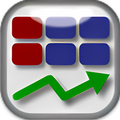# Excel Regression Analysis for Windows

• Trial version
• In English
• Version: 3

## Author's review

### Excel multiple regression analysis template with advanced statistical test results and automatic forecasting options.

The Excel Regression Analysis and Forecasting model provides a reliable basis for identifying value drivers and forecasting business and scientific data. While the regression analysis utilizes a range of commonly employed statistical measures to test the validity of the analysis, results are summarized in text for ease of use. Once relationships have been identified, forecasting can be accomplished based on a range of available methodologies. The Excel regression analysis solution provides much more functionality and more intuitive results display than the standard regression analysis provided within Excel via the Analysis Toolpak add-in.

Ease and flexibility of input, with embedded help prompts. User-friendly results display for the non-statistician. Multiple and individual regression of independent variables. Tests for statistical significance, autocorrelation, and multicollinearity. Quick forecasting process with options to employ 3rd order polynomial, 2nd order polynomial, exponential or linear trend lines on independent variables.

Advanced statistical tests are undertaken to confirm the validity of predictive relationships identified in the input data. Forecasting can be undertaken based on linear or exponential forecasts of independent variables or left blank for input of external calculated data. Compatible with all versions of Excel for Windows and Excel for Mac 2011 and 2004.

PROS

• Simple and easy to use.
• Advanced statistical testing for confident forecasting.

CONS

• Requires Microsoft Excel 97 or higher.